## The Government of India is planning to fix a hoarding board at the face of a building on the road of a busy market for awareness on COVID-19 protocol. Ram, Robert and Rahim are the three engineers who are working on this project. “A” is considered to be a person viewing the hoarding board 20 metres away from the building, standing at the edge of a pathway nearby. Ram, Robert and Rahim suggested to the firm to place the hoarding board at three different locations namely C, D and E. “C” is at the height of 10 metres from the ground level. For the viewer A, the angle of elevation of “D” is double the angle of elevation of “C” The angle of elevation of “E” is triple the angle of elevation of “C” for the same viewer. Look at the figure given and based on the above information and the following: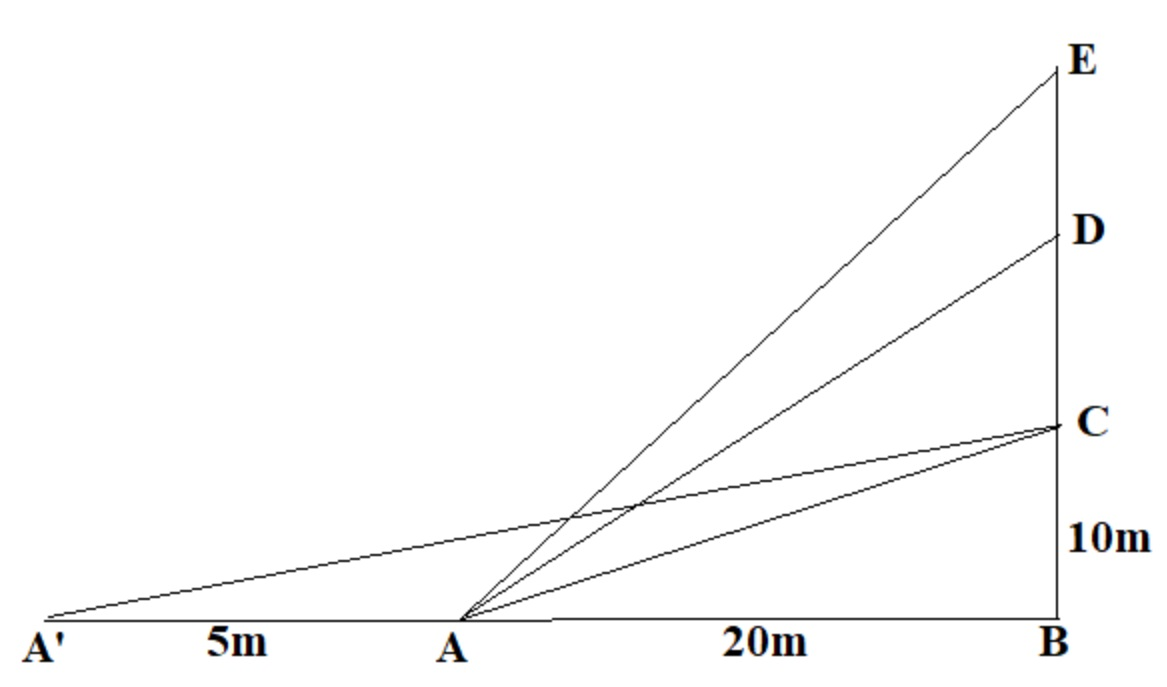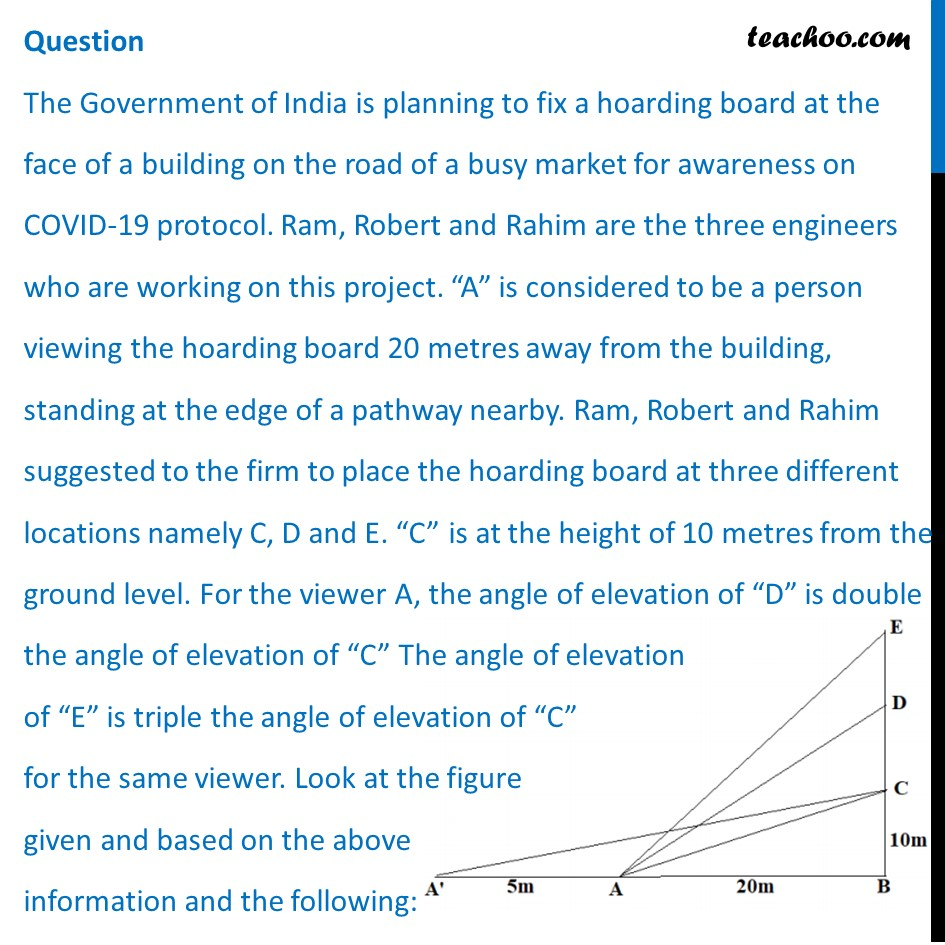## (a)  tan (-1)   (2) (b)  tan (-1)   (1/2) (c)  tan (-1)   (1) (d)  tan (-1)   (3)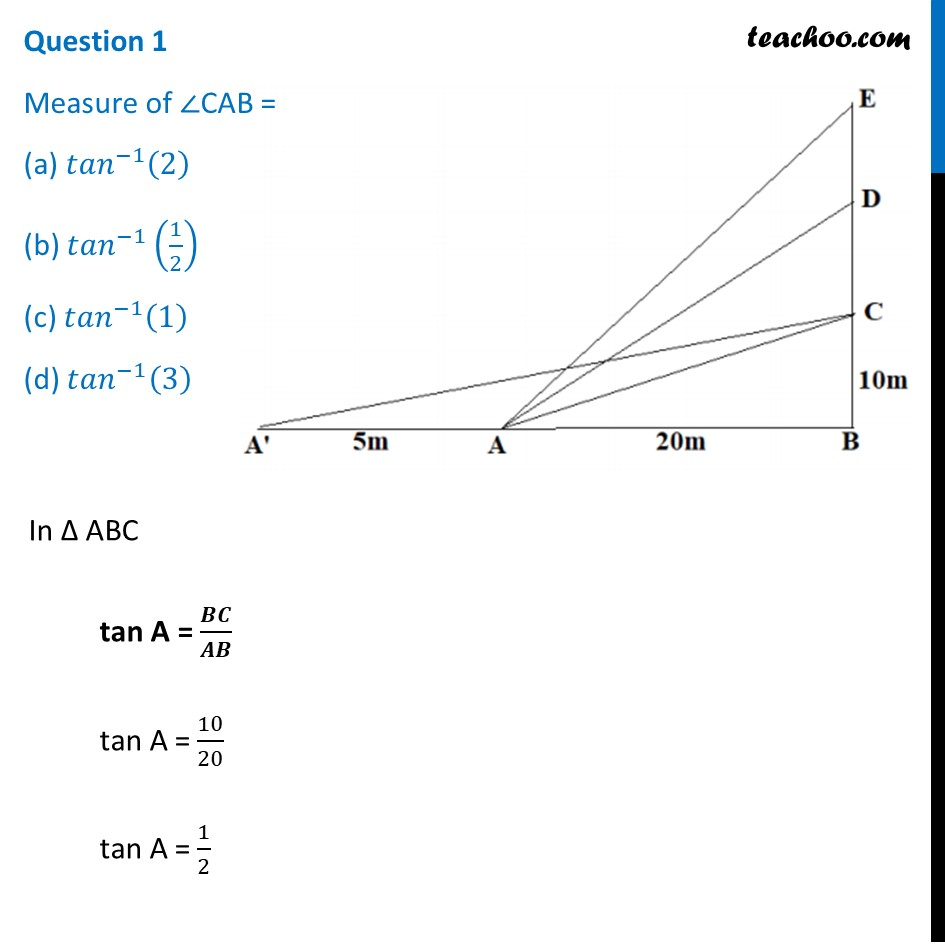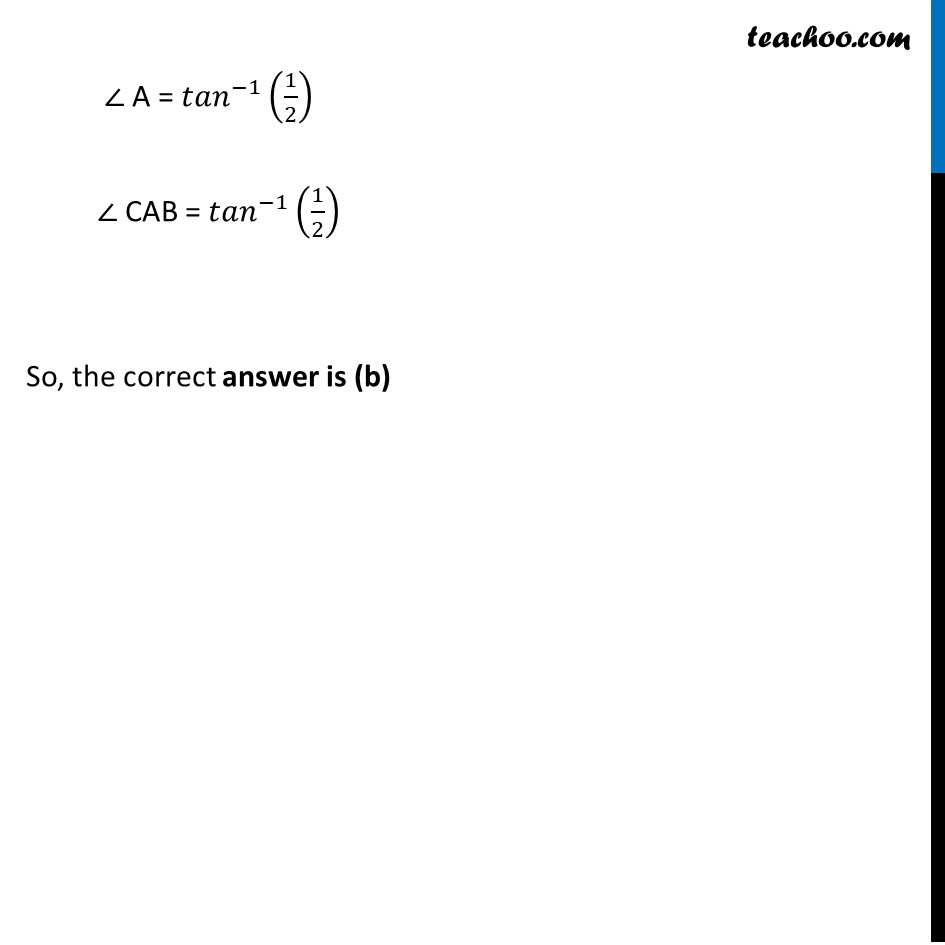## (a)  tan (-1)   (3/4) (b)  tan (-1)   (3) (c)  tan (-1)   (4/3) (d)  tan (-1)  (4)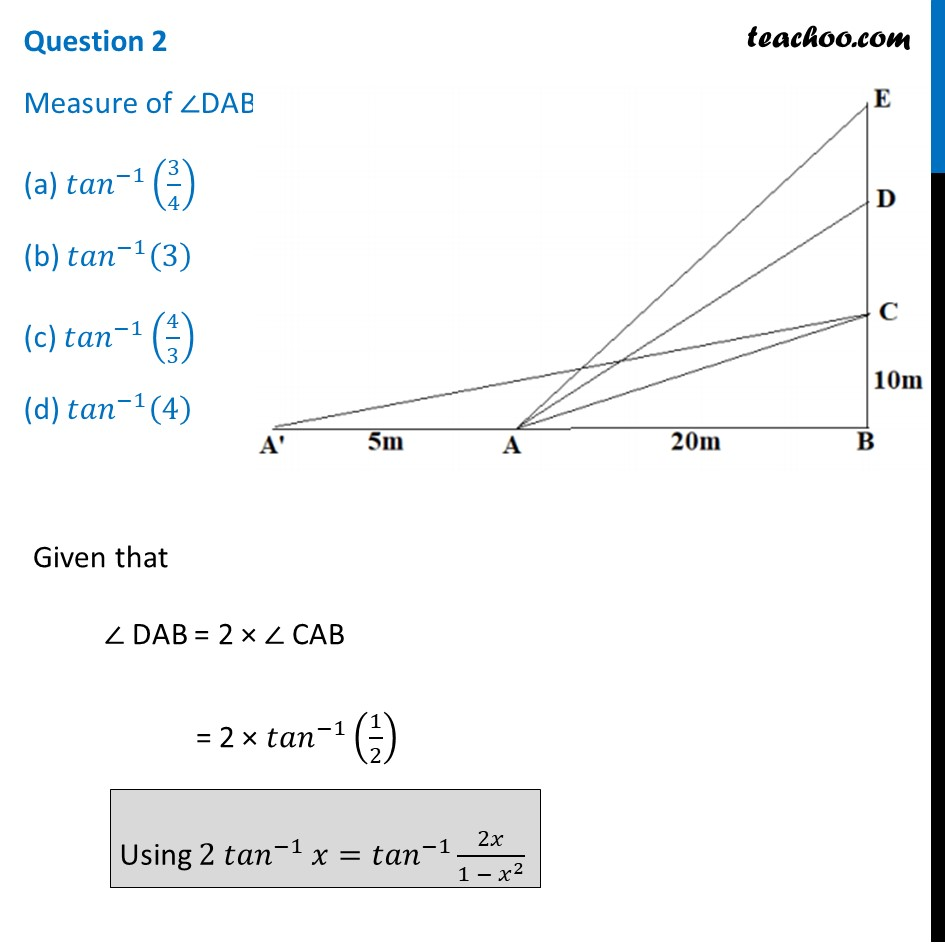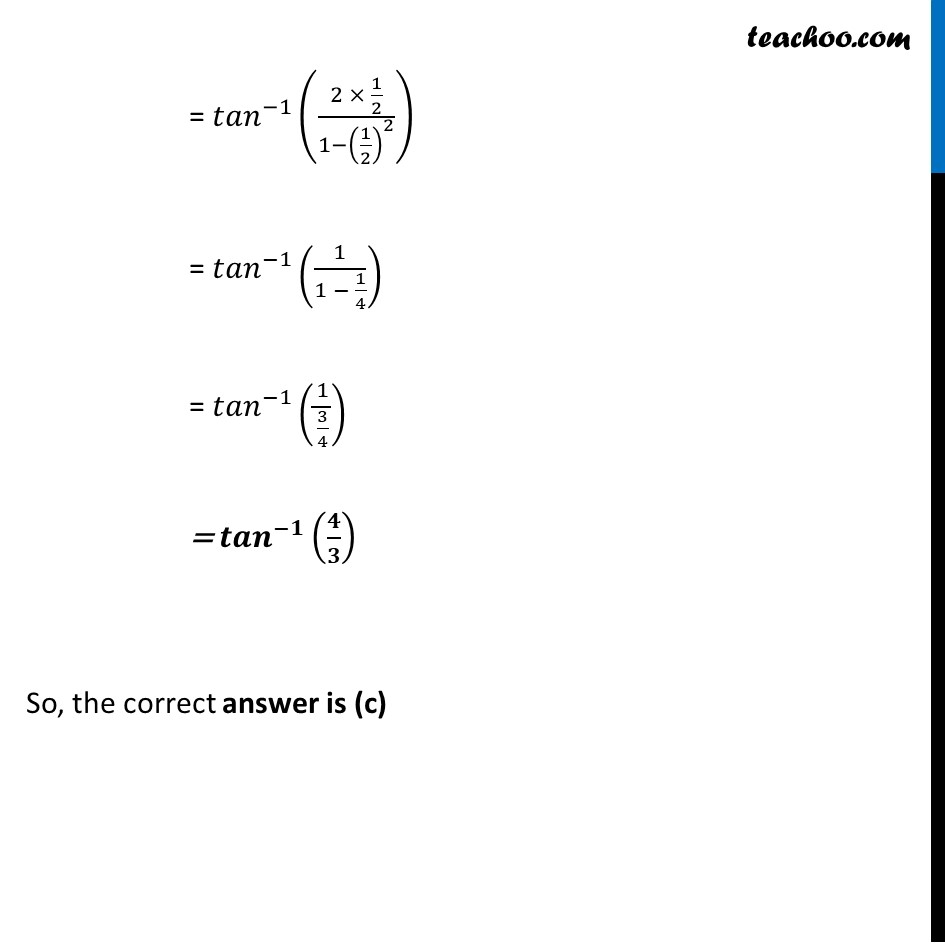## (a)  tan (-1)   (11) (b)  tan (-1)   3 (c)  tan (-1)   (2/11) (d)  tan (-1)   (11/2)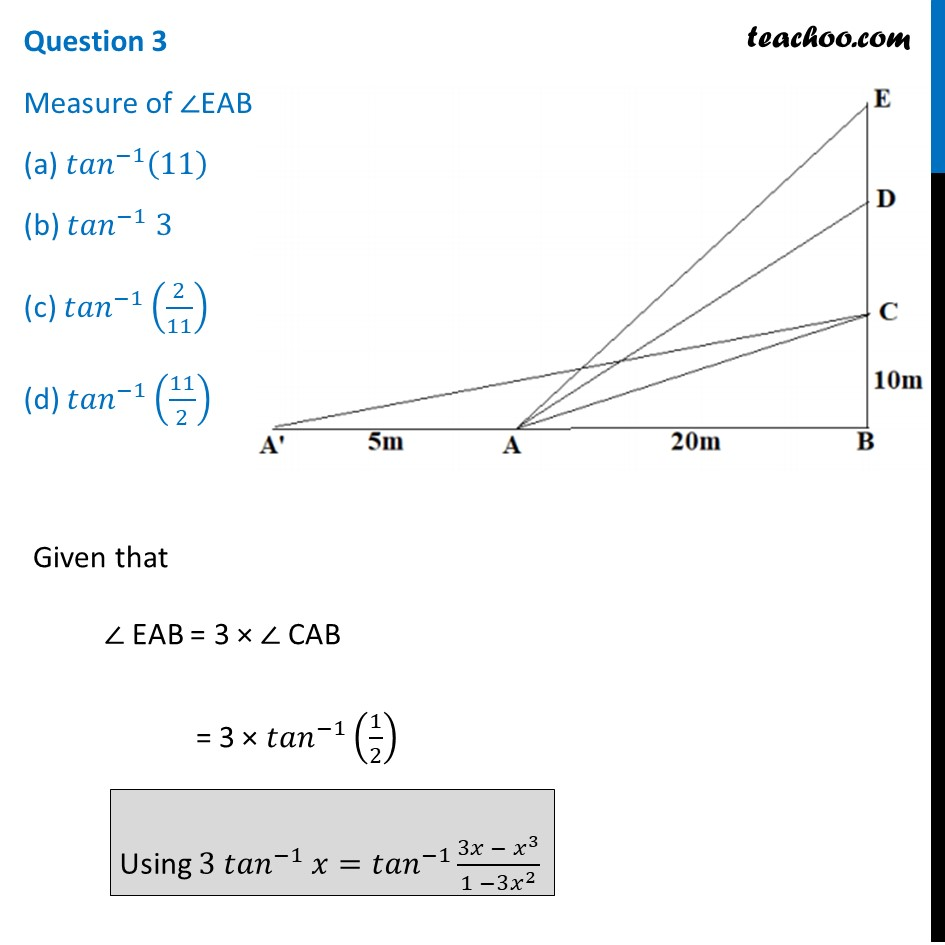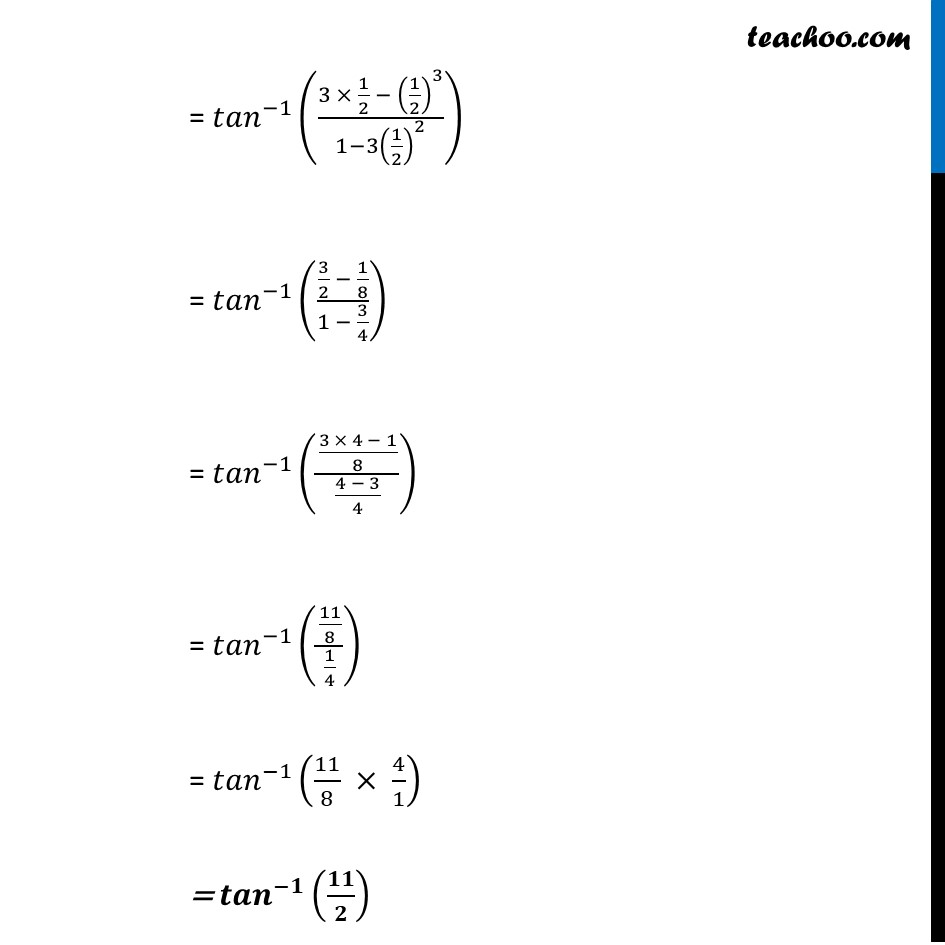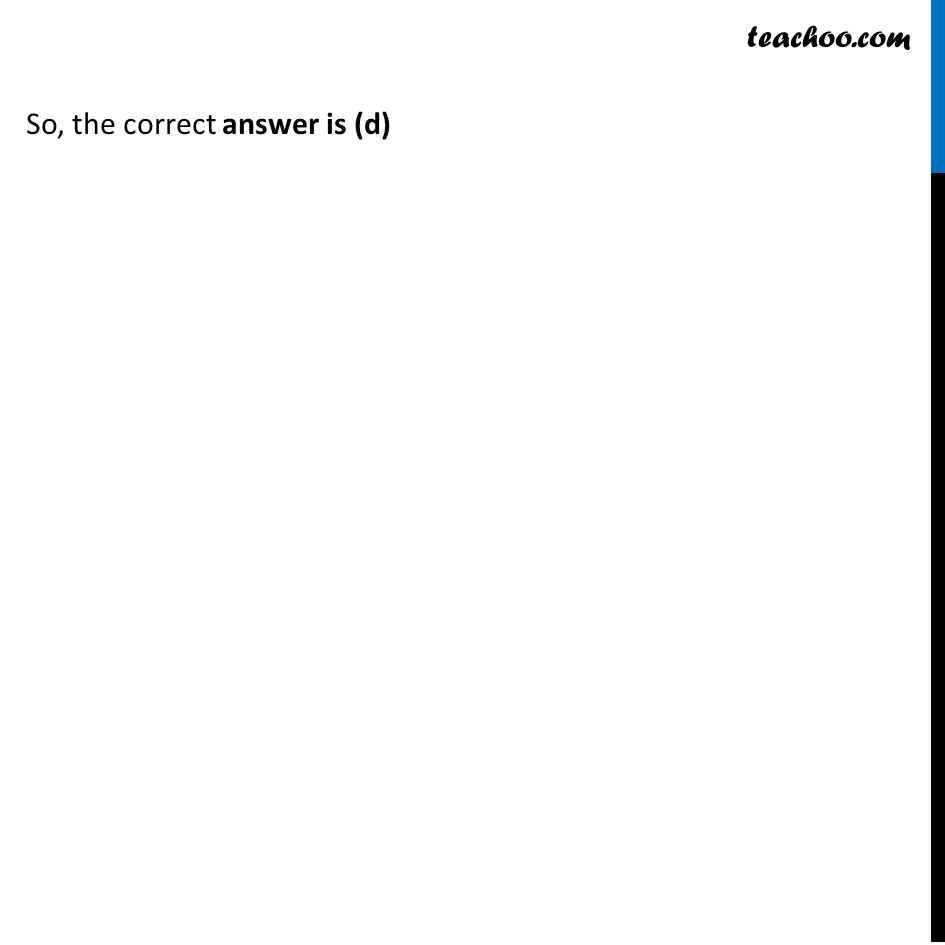## (a)  tan (-1)   (1/2) (b)  tan (-1) (1/8) (c)  tan (-1) (2/5) (d)  tan (-1) (11/21)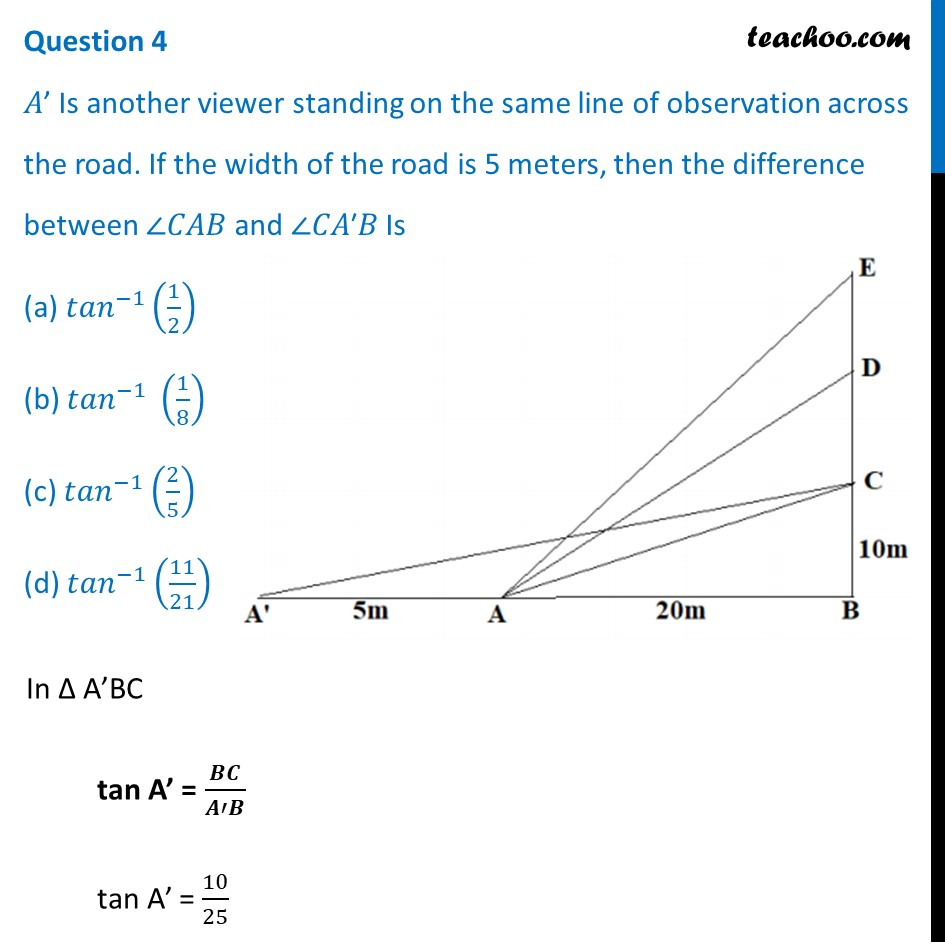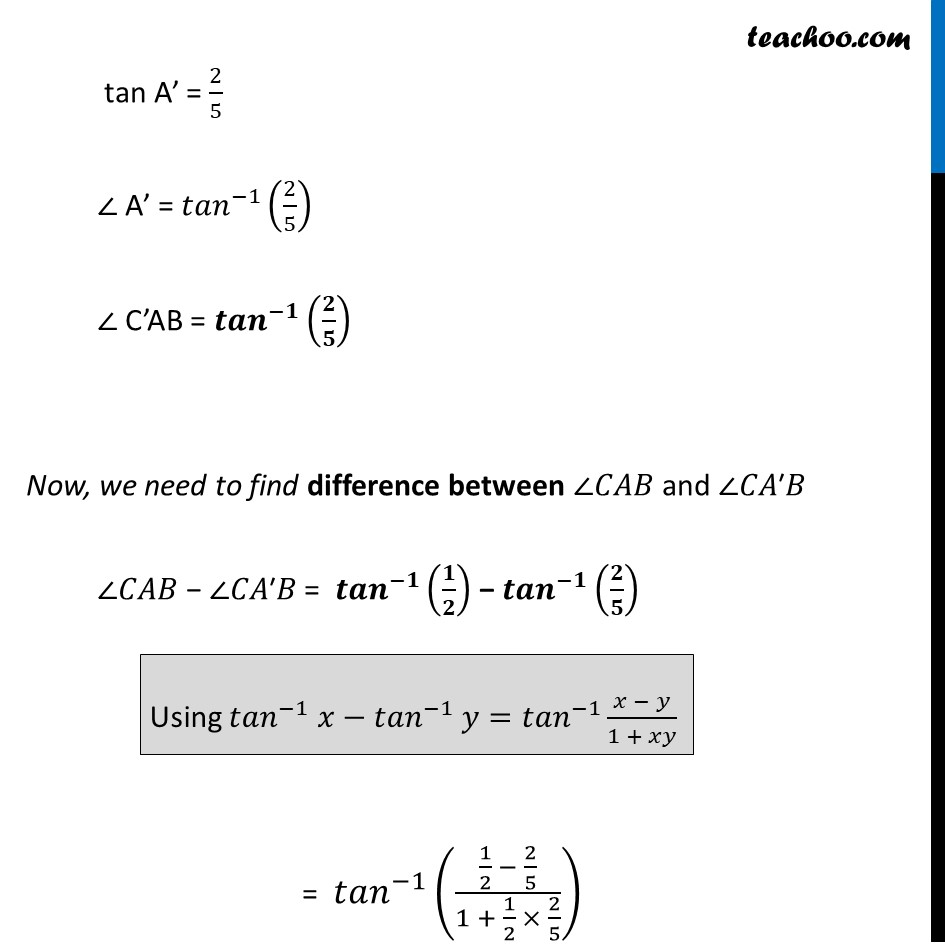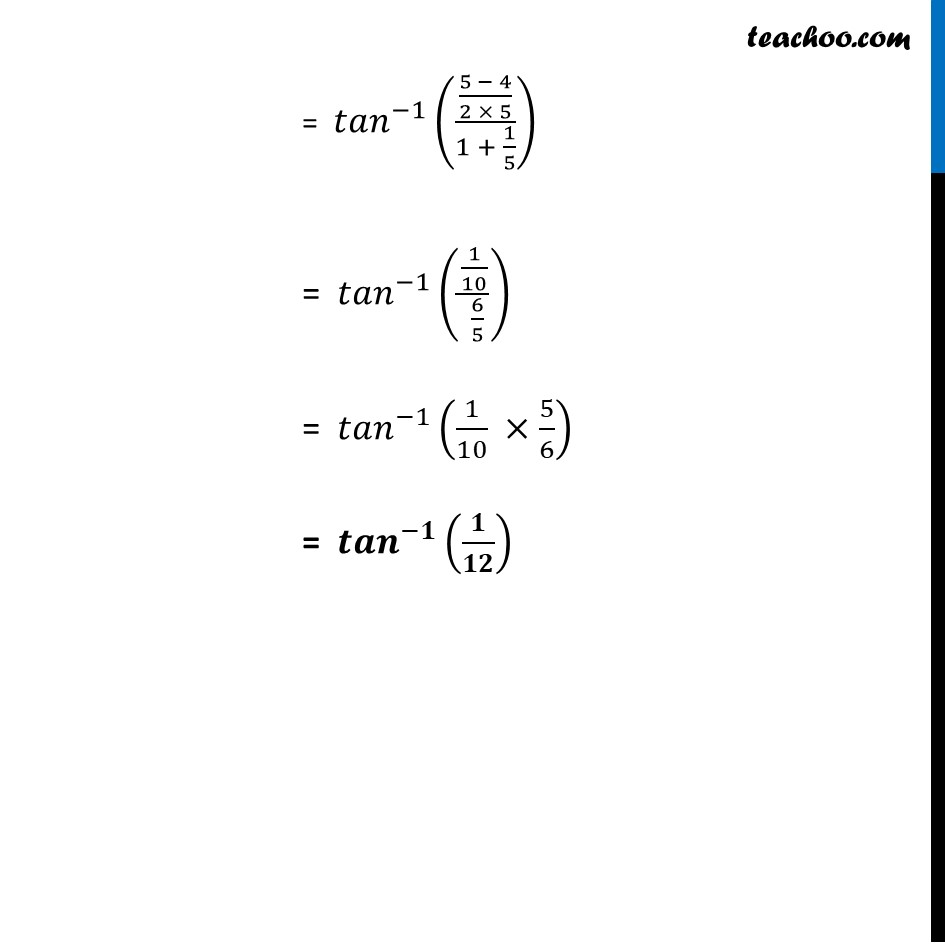Note: This answer doesn’t match with any of the options

## (a)  R+ , (-π/2, π/2) (b)  R– , (-π/2, π/2) (c)  R , (-π/2, π/2) (d)  R , (0, π/2)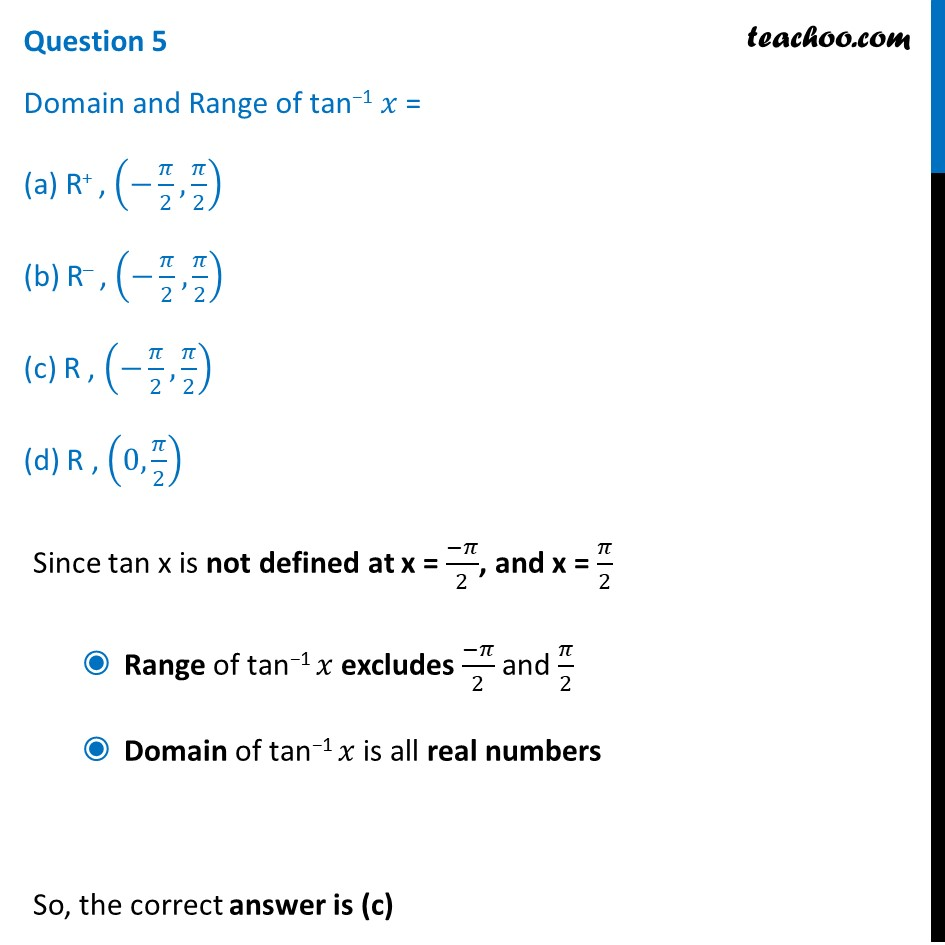1. Chapter 2 Class 12 Inverse Trigonometric Functions (Term 1)
2. Serial order wise
3. Case Based Questions (MCQ)

Transcript

The Government of India is planning to fix a hoarding board at the face of a building on the road of a busy market for awareness on COVID-19 protocol. Ram, Robert and Rahim are the three engineers who are working on this project. “A” is considered to be a person viewing the hoarding board 20 metres away from the building, standing at the edge of a pathway nearby. Ram, Robert and Rahim suggested to the firm to place the hoarding board at three different locations namely C, D and E. “C” is at the height of 10 metres from the ground level. For the viewer A, the angle of elevation of “D” is double the angle of elevation of “C” The angle of elevation of “E” is triple the angle of elevation of “C” for the same viewer. Look at the figure given and based on the above information and the following: Question 1 Measure of ∠CAB = (a) 〖𝑡𝑎𝑛〗^(−1) (2) (b) 〖𝑡𝑎𝑛〗^(−1) (1/2) (c) 〖𝑡𝑎𝑛〗^(−1) (1) (d) 〖𝑡𝑎𝑛〗^(−1) (3) In Δ ABC tan A = 𝑩𝑪/𝑨𝑩 tan A = 10/20 tan A = 1/2 ∠ A = 〖𝑡𝑎𝑛〗^(−1) (1/2) ∠ CAB = 〖𝑡𝑎𝑛〗^(−1) (1/2) So, the correct answer is (b) Question 2 Measure of ∠DAB = (a) 〖𝑡𝑎𝑛〗^(−1) (3/4) (b) 〖𝑡𝑎𝑛〗^(−1) (3) (c) 〖𝑡𝑎𝑛〗^(−1) (4/3) (d) 〖𝑡𝑎𝑛〗^(−1) (4) Given that ∠ DAB = 2 × ∠ CAB = 2 × 〖𝑡𝑎𝑛〗^(−1) (1/2) = 〖𝑡𝑎𝑛〗^(−1) ((2 × 1/2)/(1−(1/2)^2 )) = 〖𝑡𝑎𝑛〗^(−1) (1/(1 − 1/4)) = 〖𝑡𝑎𝑛〗^(−1) (1/(3/4)) = 〖𝒕𝒂𝒏〗^(−𝟏) (𝟒/𝟑) So, the correct answer is (c) Question 3 Measure of ∠EAB = (a) 〖𝑡𝑎𝑛〗^(−1) (11) (b) 〖𝑡𝑎𝑛〗^(−1) 3 (c) 〖𝑡𝑎𝑛〗^(−1) (2/11) (d) 〖𝑡𝑎𝑛〗^(−1) (11/2) Given that ∠ EAB = 3 × ∠ CAB = 3 × 〖𝑡𝑎𝑛〗^(−1) (1/2) Using 3 〖𝑡𝑎𝑛〗^(−1) 𝑥=〖𝑡𝑎𝑛〗^(−1) (3𝑥 − 𝑥^3)/(1 −3𝑥^2 ) = 〖𝑡𝑎𝑛〗^(−1) ((3 × 1/2 − (1/2)^3)/(1−〖3(1/2)〗^2 )) = 〖𝑡𝑎𝑛〗^(−1) ((3/2 − 1/8)/(1 − 3/4)) = 〖𝑡𝑎𝑛〗^(−1) (((3 × 4 − 1)/8)/((4 − 3)/4)) = 〖𝑡𝑎𝑛〗^(−1) ((11/8)/(1/4)) = 〖𝑡𝑎𝑛〗^(−1) (11/8 × 4/1) = 〖𝒕𝒂𝒏〗^(−𝟏) (𝟏𝟏/𝟐) So, the correct answer is (d) Question 4 𝐴’ Is another viewer standing on the same line of observation across the road. If the width of the road is 5 meters, then the difference between ∠𝐶𝐴𝐵 and ∠𝐶𝐴′𝐵 Is (a) 〖𝑡𝑎𝑛〗^(−1) (1/2) (b) 〖𝑡𝑎𝑛〗^(−1) (1/8) (c) 〖𝑡𝑎𝑛〗^(−1) (2/5) (d) 〖𝑡𝑎𝑛〗^(−1) (11/21) In Δ A’BC tan A’ = 𝑩𝑪/𝑨′𝑩 tan A’ = 10/25 tan A’ = 2/5 ∠ A’ = 〖𝑡𝑎𝑛〗^(−1) (2/5) ∠ C’AB = 〖𝒕𝒂𝒏〗^(−𝟏) (𝟐/𝟓) Now, we need to find difference between ∠𝐶𝐴𝐵 and ∠𝐶𝐴′𝐵 ∠𝐶𝐴𝐵 − ∠𝐶𝐴′𝐵 = 〖𝒕𝒂𝒏〗^(−𝟏) (𝟏/𝟐) − 〖𝒕𝒂𝒏〗^(−𝟏) (𝟐/𝟓) = 〖𝑡𝑎𝑛〗^(−1) ((1/2 − 2/5)/(1 + 1/2 × 2/5)) = 〖𝑡𝑎𝑛〗^(−1) (((5 − 4)/(2 × 5))/(1 + 1/5)) = 〖𝑡𝑎𝑛〗^(−1) ((1/10)/( 6/5)) So, the correct answer is (b) = 〖𝑡𝑎𝑛〗^(−1) (((5 − 4)/(2 × 5))/(1 + 1/5)) = 〖𝑡𝑎𝑛〗^(−1) ((1/10)/( 6/5)) = 〖𝑡𝑎𝑛〗^(−1) (1/10 ×5/6) = 〖𝒕𝒂𝒏〗^(−𝟏) (𝟏/𝟏𝟐) Question 5 Domain and Range of tan−1 𝑥 = (a) R+ , (−𝜋/2,𝜋/2) (b) R– , (−𝜋/2,𝜋/2) (c) R , (−𝜋/2,𝜋/2) (d) R , (0,𝜋/2) Since tan x is not defined at x = (−𝜋)/2, and x = 𝜋/2 Range of tan−1 𝑥 excludes (−𝜋)/2 and 𝜋/2 Domain of tan−1 𝑥 is all real numbers So, the correct answer is (c)

Case Based Questions (MCQ)